Next: Implementation Up: Weyl filter Previous: Weyl filter   Contents

Mathematical background

Let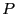be a polynomial and be maxroot the maximal modulus of the root of. Fromwe may derive a the unitary polynomial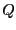such that the roots ofhave a modulus lower or equal to 1 and if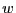is a root ofthen maxrootis a root of.

Let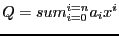which may also be written as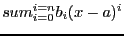where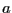is some fixed point.

Let a range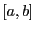for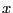and let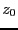be the mid point of the range. We consider the square in the complex plane centered atand whose edge length is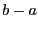. Let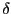be the length of the half-diagonal of this square. If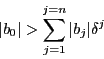then the polynomial has no root in the square . This procedure may be used for univariate polynomial, with polynomial with interval coefficients or with parametric poynomial. For example it is very efficient for solging the Wilkinson polynomial.Next: Implementation Up: Weyl filter Previous: Weyl filter   Contents
Jean-Pierre Merlet 2012-12-20